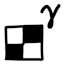#Gamma-aware image dithering

## Introduction

When converting an image to a lower bit depth and adding dither to reduce banding, it’s important to take the display gamma into account. For example on the 8-bit scale that ranges from 0 to 255, on typical displays designed for gamma 2.2 or sRGB, the midpoint value 127.5 is not 50% the brightness of 255 – it’s actually about 22% of the brightness. The implication is that if we approximate the gray value 127.5 by alternating between white and black pixels, then we need 22% of the pixels to be white, not 50% of them. (For the purpose of this discussion, we will refer gamma 2.2 and sRGB interchangeably.)

## Demo

This example image starts at 8 bits per channel (256 possible values), and is quantized down to 2 bits per channel (4 possible values). Notice how the naive dithering method inappropriately increases the brightness of the image (in the river and grass on the bottom side), whereas the gamma-aware dithering faithfully preserves the average image brightness. In fact if you squint, you will see that the gamma-aware dithered image looks essentially the same as the original image, proving that the dithering is working properly.

Note that the dithered images must be displayed at 1:1 zoom without any resampling, otherwise the resampler (which is almost never gamma-aware) will destroy the integrity of the image.

## Algorithms

The naive way of doing dithering is like this (pseudocode):

```accumulatedError = 0  # In gamma/sRGB space
for (x,y) from (0,0) to (w-1,h-1):  # In some reasonable scan order
outputPixel[x,y] = quantize(inputPixel[x,y] - accumulatedError)
accumulatedError += outputPixel[x,y] - inputPixel[x,y]```

The function `quantize` means to select a valid output value that best approximates the input argument.

The correct way to do dithering is like this (pseudocode):

```accumulatedError = 0  # In linear space
for (x,y) from (0,0) to (w-1,h-1):  # In some reasonable scan order
inputLinear = gammaToLinear(inputPixel[x,y])
outputPixel[x,y] = quantizeLinearToGamma(inputLinear - accumulatedError)
accumulatedError += gammaToLinear(outputPixel[x,y]) - inputLinear```

Here, `quantizeLinearToGamma` should select the output value that is closest to the input argument in linear space, not necessarily in sRGB/gamma space. In other words, we minimize `abs(gammaToLinear(quantizeLinearToGamma(z)) - z)` instead of `abs(quantizeLinearToGamma(z) - linearToGamma(z))`.

## Source code

Usage: `java GammaAwareImageDithering Input.png/bmp naive/srgb Output.png`

This program converts the input image (assumed to be 8 bits per channel) to a heavily dithered output image that is 2 bits per channel. This proof-of-concept program is featureless, so you’ll need to play around with the code to modify the output bit depth, change the dithering algorithm, etc.

## Notes

• The simple algorithm presented above merely dumps the approximation error to the next pixel to the right. It’s easy to adapt this algorithm for use in better error-diffusion methods like Floyd–Steinberg dithering. It’s also possible to implement gamma-aware ordered dithering with some careful thought.

• If the input image values are already in linear space (such as purely computer-generated graphics, especially with anti-aliasing), then we can skip the initial conversion from sRGB to linear.

• The Golden Gate Bridge photo is by Denis Cappellin, licensed under Creative Commons.